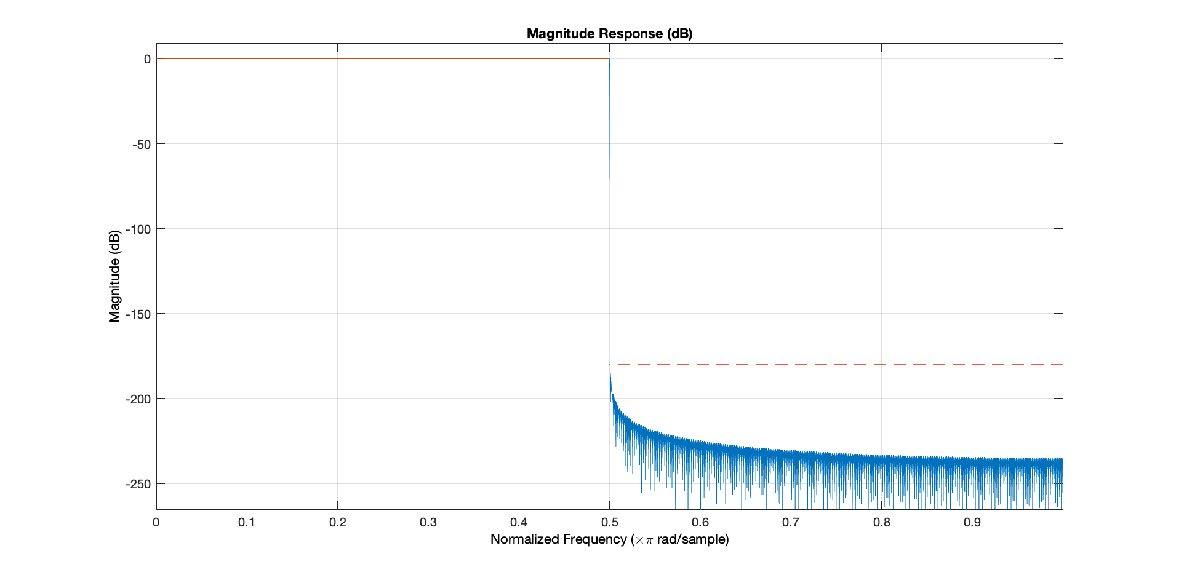# FIR Halfband Filter Design

This example shows how to design FIR halfband filters. Halfband filters are widely used in multirate signal processing applications when interpolating or decimating by a factor of two. Halfband filters are implemented efficiently in polyphase form because approximately half of the halfband filter coefficients are equal to zero.

Halfband filters have two important characteristics:

• The passband and stopband ripples must be the same.

• The passband-edge and the stopband-edge frequencies are equidistant from the halfband frequency $\frac{\mathrm{Fs}}{4}$ (or $\frac{\pi }{2}$ rad/sample in normalized frequency).

### Obtaining the Halfband Coefficients

The `firhalfband` function returns the coefficients of an FIR halfband equiripple filter. As a simple example, consider a halfband filter dealing with data sampled at 96 kHz and has a passband frequency of 22 kHz.

```Fs = 96e3; Fp = 22e3; N = 100; num = firhalfband(N,Fp/(Fs/2)); zerophase(num,1,linspace(0,Fs/2,512),Fs);```By zooming into the response, you can verify that the passband and stopband peak-to-peak ripples are the same. Also there is symmetry about the $\frac{\mathrm{Fs}}{4}$ (24 kHz) point. The passband extends up to 22 kHz as specified and the stopband begins at 26 kHz. We can also verify that every other coefficient is equal to zero by looking at the impulse response. This makes the filter very efficient to implement for interpolation or decimation by a factor of 2.

```fvt = fvtool(num,Fs=Fs); fvt.Analysis = "impulse";```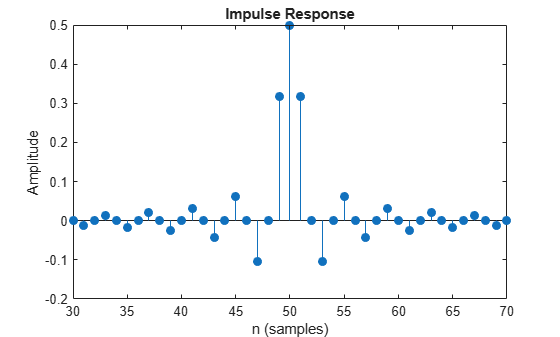### `dsp.FIRHalfbandInterpolator` and `dsp.FIRHalfbandDecimator`

The `firhalfband` function provides several other design options. However, using `dsp.FIRHalfbandInterpolator` and `dsp.FIRHalfbandDecimator` System objects is recommended when working with streaming data. These two System objects not only design the coefficients, but also provide efficient polyphase implementation. They support filtering double, single precision floating-point data as well as fixed-point data. They also support C and HDL code generation as well as optimized ARM® Cortex® M and ARM® Cortex® A code generation.

```halfbandInterpolator = dsp.FIRHalfbandInterpolator(SampleRate=Fs,... Specification="Filter order and transition width",... FilterOrder=N,TransitionWidth=4000); fvt = fvtool(halfbandInterpolator,Fs=2*Fs); %#ok<NASGU> ```In order to perform the interpolation, use the `dsp.FIRHalfbandInterpolator` System object™. Because this is a multirate filter, it is important to define what is meant by the sample rate. For this and all other System objects, the sample rate refers to the sample rate of the input signal. However, FVTool defines the sample rate as the rate at which the filter is running. In the case of interpolation, you upsample and then filter (conceptually), therefore the sample rate of FVTool needs to be specified as $2\mathrm{Fs}$ because of the upsampling by 2.

```FrameSize = 256; scope = spectrumAnalyzer(SampleRate=2*Fs); sine1 = dsp.SineWave(Frequency=10e3,SampleRate=Fs,... SamplesPerFrame=FrameSize); sine2 = dsp.SineWave(Frequency=20e3,SampleRate=Fs,... SamplesPerFrame=FrameSize); tic while toc < 10 x = sine1() + sine2() + 0.01.*randn(FrameSize,1); % 96 kHz y = halfbandInterpolator(x); % 192 kHz scope(y); end release(scope);```Notice that the spectral replicas are attenuated by about 40 dB which is roughly the attenuation provided by the halfband filter. You can obtain a plot with the interpolated samples overlaid on the input samples by compensating for the group-delay of the filter. Notice that the input samples remain unchanged at the output of the filter. This is because one of the polyphase branches of the halfband filter is a pure delay branch which does not change the input samples.

```grpDel = 50; n = 0:2:511; stem(n(1:end-grpDel/2),x(1:end-grpDel/2),"k","filled") hold on nu = 0:511; stem(nu(1:end-grpDel),y(grpDel+1:end)) legend("Input samples","Interpolated samples")```In the case of decimation, the sample rate specified in `dsp.FIRHalfbandDecimator` corresponds to the sample rate of the filter, since the object filters and then downsamples (conceptually). So for decimators, the$\text{\hspace{0.17em}}\mathrm{Fs}$ specified in FVTool does not need to be multiplied by any factor.

```FrameSize = 256; FsIn = 2*Fs; halfbandDecimator = dsp.FIRHalfbandDecimator(SampleRate=FsIn,... Specification="Filter order and transition width",... FilterOrder=N,TransitionWidth=4000); fvt = fvtool(halfbandDecimator,Fs=FsIn);%#ok<NASGU> scope = spectrumAnalyzer(SampleRate=Fs); sine1 = dsp.SineWave(Frequency=10e3,SampleRate=Fs,... SamplesPerFrame=FrameSize); sine2 = dsp.SineWave(Frequency=20e3,SampleRate=Fs,... SamplesPerFrame=FrameSize); tic while toc < 10 x = sine1() + sine2() + 0.01.*randn(FrameSize,1); % 96 kHz y = halfbandInterpolator(x); % 192 kHz xd = halfbandDecimator(y); % 96 kHz scope(xd); end````release(scope);`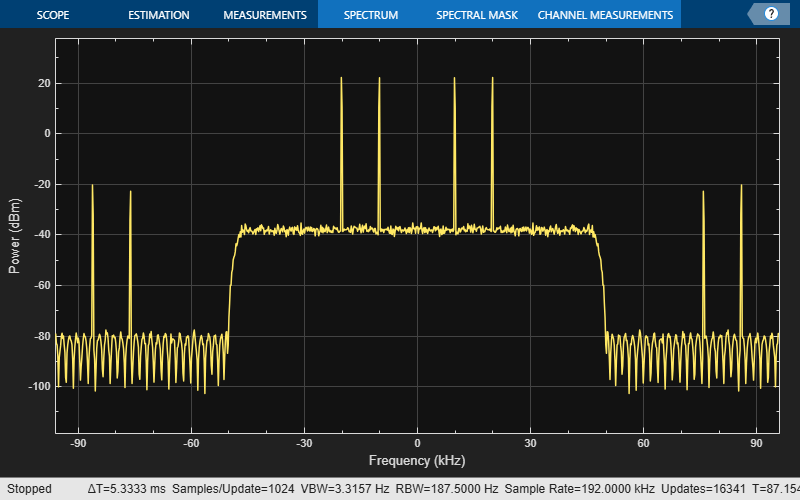### Obtaining the Filter Coefficients

The filter coefficients can be extracted from the interpolator/decimator by using the `tf` function.

`num = tf(halfbandInterpolator); % Or num = tf(halfbandDecimator);`

### Using Different Design Specifications

Instead of specifying the filter order and transition width, you can design a minimum-order filter that provides a given transition width as well as a given stopband attenuation.

```Ast = 80; % 80 dB halfbandInterpolator = dsp.FIRHalfbandInterpolator(SampleRate=Fs,... Specification="Transition width and stopband attenuation",... StopbandAttenuation=Ast,TransitionWidth=4000); fvtool(halfbandInterpolator,Fs=2*Fs);```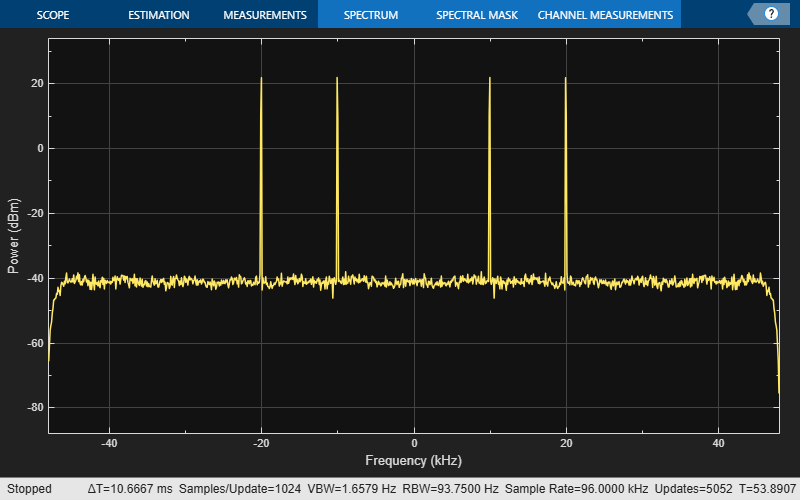Notice that as with all interpolators, the passband gain in absolute units is equal to the interpolation factor (2 in the case of halfbands). This corresponds to a passband gain of 6.02 dB.

It is also possible to specify the filter order and the stopband attenuation.

```halfbandDecimator = dsp.FIRHalfbandDecimator(SampleRate=Fs,... Specification="Filter order and stopband attenuation",... StopbandAttenuation=Ast,FilterOrder=N); fvtool(halfbandDecimator,Fs=Fs);```Unlike interpolators, decimators have a gain of 1 (0 dB) in the passband.

### Using Halfband Filters for Filter Banks

Halfband interpolators and decimators can be used to efficiently implement synthesis/analysis filter banks. The halfband filters shown so far have all been lowpass filters. With a single extra adder, it is possible to obtain a highpass response in addition to the lowpass response and use the two responses for the filter bank implementation.

The following code simulates a quadrature mirror filter (QMF) bank. An 8 kHz signal consisting of 1 kHz and 3 kHz sine waves is separated into two 4 kHz signals using a lowpass/highpass halfband decimator. The lowpass signal retains the 1 kHz sine wave while the highpass signal retains the 3 kHz sine wave (which is aliased to 1 kHz after downsampling). The signals are then merged back together with a synthesis filter bank using a halfband interpolator. The highpass branch upconverts the aliased 1 kHz sine wave back to 3 kHz. The interpolated signal has an 8 kHz sample rate.

```Fs1 = 8000; % Units = Hz Spec = "Filter order and transition width"; Order = 52; TW = 4.1e2; % Units = Hz % Construct FIR Halfband Interpolator halfbandInterpolator = dsp.FIRHalfbandInterpolator( ... Specification=Spec,... FilterOrder=Order,... TransitionWidth=TW,... SampleRate=Fs1/2,... FilterBankInputPort=true); % Construct FIR Halfband Decimator halfbandDecimator = dsp.FIRHalfbandDecimator( ... Specification=Spec,... FilterOrder=Order,... TransitionWidth=TW,... SampleRate=Fs1); % Input f1 = 1000; f2 = 3000; InputWave = dsp.SineWave(Frequency=[f1,f2],SampleRate=Fs1,... SamplesPerFrame=1024,Amplitude=[1 0.25]); % Construct Spectrum Analyzer object to view the input and output scope = spectrumAnalyzer(SampleRate=Fs1,... PlotAsTwoSidedSpectrum=false,ShowLegend=true,... YLimits=[-120 30],... Title="Input Signal and Output Signal of Quadrature Mirror Filter",... ChannelNames={"Input","Output"}); %#ok<CLARRSTR> tic while toc < 10 Input = sum(InputWave(),2); NoisyInput = Input+(10^-5)*randn(1024,1); [Lowpass,Highpass] = halfbandDecimator(NoisyInput); Output = halfbandInterpolator(Lowpass,Highpass); scope([NoisyInput,Output]); end release(scope);```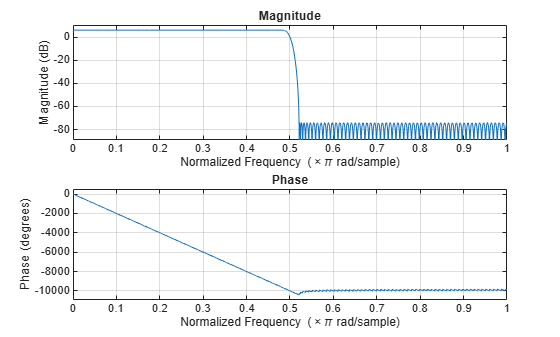### Advanced Design Options: Specifying Different Design Algorithms

All designs presented so far have been optimal equiripple designs. `dsp.FIRHalfbandDecimator` and `dsp.FIRHalfbandInterpolator` System objects can also design their filters using the Kaiser window method.

```Fs = 44.1e3; N = 90; TW = 1000; equirippleHBFilter = dsp.FIRHalfbandInterpolator(DesignMethod="Equiripple",... Specification="Filter order and transition width",... SampleRate=Fs,... TransitionWidth=TW,... FilterOrder=N); kaiserHBFilter = dsp.FIRHalfbandInterpolator(DesignMethod="Kaiser",... Specification="Filter order and transition width",... SampleRate=Fs,... TransitionWidth=TW,... FilterOrder=N); ```

You can compare the designs with FVTool. The two designs allow for tradeoffs between minimum stopband attenuation and larger overall attenuation.

```fvt = fvtool(equirippleHBFilter,kaiserHBFilter,Fs=2*Fs); legend(fvt,"Equiripple design","Kaiser-window design")```If you use the`fdesign.interpolator` and `fdesign.decimator` objects, other design algorithms, such as Least-square linear-filter FIR filter design are available. To determine the list of available design methods for a given filter specification object, use the `designmethods` function.

```filtSpecs = fdesign.interpolator(2,"halfband","N,TW",N,TW/Fs); designmethods(filtSpecs,"FIR");```
```FIR Design Methods for class fdesign.interpolator (N,TW): equiripple firls kaiserwin ```

### Controlling the Stopband Attenuation

Alternatively, one can specify the order and the stopband attenuation. This allows for tradeoffs between overall stopband attenuation and transition width.

```Ast = 60; % Minimum stopband attenuation equirippleHBFilter = dsp.FIRHalfbandInterpolator(DesignMethod="Equiripple",... Specification="Filter order and stopband attenuation",... SampleRate=Fs,... StopbandAttenuation=Ast,... FilterOrder=N); kaiserHBFilter = dsp.FIRHalfbandInterpolator(DesignMethod="Kaiser",... Specification="Filter order and stopband attenuation",... SampleRate=Fs,... StopbandAttenuation=Ast,... FilterOrder=N); fvt = fvtool(equirippleHBFilter,kaiserHBFilter,Fs=2*Fs); legend(fvt,"Equiripple design","Kaiser-window design")```### Minimum-Order Designs

Kaiser window designs can also be used in addition to equiripple designs when designing a filter of the minimum-order necessary to meet the design specifications. The actual order for the Kaiser window design is larger than that needed for the equiripple design, but the overall stopband attenuation is better in return.

```Fs = 44.1e3; TW = 1000; % Transition width Ast = 60; % 60 dB minimum attenuation in the stopband equirippleHBFilter = dsp.FIRHalfbandDecimator(DesignMethod="Equiripple",... Specification="Transition width and stopband attenuation",... SampleRate=Fs,... TransitionWidth=TW,... StopbandAttenuation=Ast); kaiserHBFilter = dsp.FIRHalfbandDecimator(DesignMethod="Kaiser",... Specification="Transition width and stopband attenuation",... SampleRate=Fs,... TransitionWidth=TW,... StopbandAttenuation=Ast); fvt = fvtool(equirippleHBFilter,kaiserHBFilter); legend(fvt,"Equiripple design","Kaiser-window design")```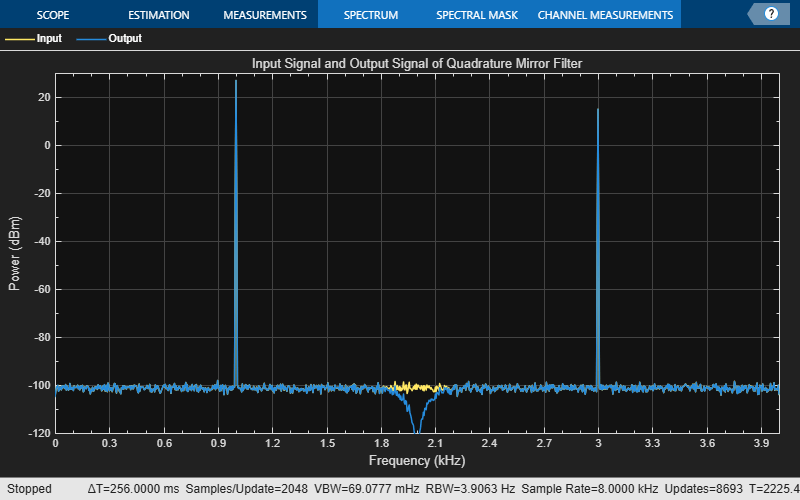### Automatic Choice of Filter Design Technique

In addition to `"Equiripple"` and `"Kaiser"`, the `DesignMethod` property of `dsp.FIRHalfbandDecimator` and `dsp.FIRHalfbandInterpolator S`ystem objects can also be specified as `"Auto"`. When `DesignMethod` is set to `"Auto"`, the filter design method is chosen automatically by the object based on the filter design parameters.

```Fs = 44.1e3; TW = 1000; % Transition width Ast = 60; % 60 dB minimum attenuation in the stopband autoHBFilter = dsp.FIRHalfbandDecimator(DesignMethod="Auto",... Specification="Transition width and stopband attenuation",... SampleRate=Fs,... TransitionWidth=TW,... StopbandAttenuation=Ast); fvt = fvtool(autoHBFilter); legend(fvt,"DesignMethod = Auto");```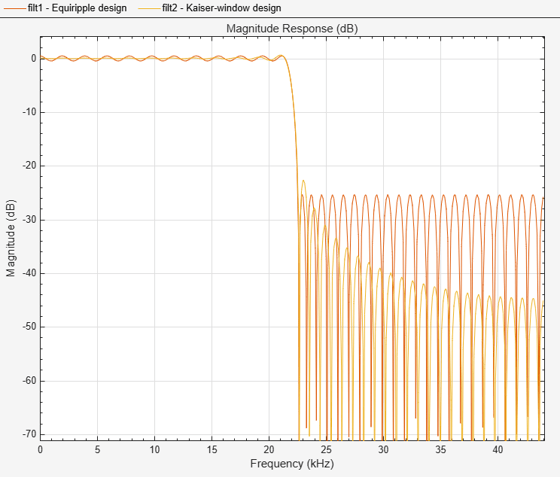For the above filter specifications, you can observe from the magnitude response that the System object designs an equiripple filter. If the design constraints are very tight such as a very high stopband attenuation or a very narrow transition width, then the algorithm automatically chooses the Kaiser window method. The Kaiser window method is optimal to design filters with very tight specifications. However, if the design constraints are not tight, then the algorithm performs equiripple design.

The following illustrates a case where the filter specifications are too tight to perform equiripple design. The `DesignMethod` property of the object is set to `"Equiripple"`. Hence the object attempts to design the filter using equiripple characteristics and the design fails to converge, resulting in warnings generated about convergence.

```Fs = 192e3; TW = 100; % Transition width Ast = 180; % 180 dB minimum attenuation in the stopband equirippleHBFilter = dsp.FIRHalfbandDecimator(DesignMethod="Equiripple",... TransitionWidth=TW,... StopbandAttenuation=Ast,... SampleRate=Fs); fvt = fvtool(equirippleHBFilter);```
```Warning: Final filter order of 10448 is probably too high to optimally meet the constraints. ```
```Warning: Design is not converging. Number of iterations was 5 1) Check the resulting filter using freqz. 2) Check the specifications. 3) Filter order may be too large or too small. 4) For multiband filters, try making the transition regions more similar in width. If err is very small, filter order may be too high ```
`legend(fvt,"DesignMethod = Equiripple");`In this case, it is possible to design a filter that converges in design by setting the `DesignMethod` property to "`Auto"` or "`Kaiser"`, and the object designs the halfband filter using the Kaiser window method.

```Fs = 192e3; TW = 100; % Transition width Ast = 180; % 180 dB minimum attenuation in the stopband autoHBFilter = dsp.FIRHalfbandDecimator(DesignMethod="Auto",... TransitionWidth=TW,... StopbandAttenuation=Ast,... SampleRate=Fs); fvt = fvtool(autoHBFilter); legend(fvt,"DesignMethod = Auto");```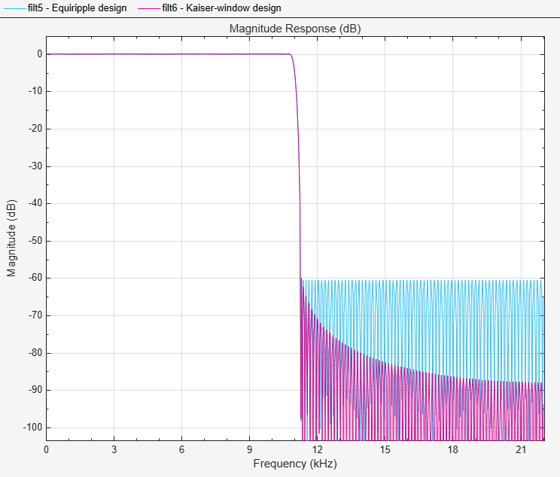### Equiripple Designs with Increasing Stopband Attenuation

Using the `fdesign.interpolator` and `fdesign.decimator `objects, you can also modify the shape of the stopband in equiripple design by specifying the optional `"StopbandShape" `argument of the `design` function.

```Fs = 44.1e3; TW = 1000/(Fs/2); % Transition width Ast = 60; % 60 dB minimum attenuation in the stopband filtSpecs = fdesign.decimator(2,"halfband","TW,Ast",TW,Ast); equirippleHBFilter1 = design(filtSpecs,"equiripple",... StopbandShape="1/f",StopbandDecay=4,SystemObject=true); equirippleHBFilter2 = design(filtSpecs,"equiripple",... StopbandShape="linear",StopbandDecay=53.333,SystemObject=true); fvt = fvtool(equirippleHBFilter1,equirippleHBFilter2,... Fs=Fs); legend(fvt,"Stopband decaying as (1/f)^4","Stopband decaying linearly")```### Highpass Halfband Filters

A highpass halfband filter can be obtained from a lowpass halfband filter by changing the sign of every second coefficient. Alternatively, one can directly design a highpass halfband by setting the `Type` property of the `fdesign.decimator` object to `"Highpass`".

```filtSpecs = fdesign.decimator(2,"halfband",... "TW,Ast",TW,Ast,Type="Highpass"); halfbandHPFilter = design(filtSpecs,"equiripple",... StopbandShape="linear",StopbandDecay=53.333,SystemObject=true); fvt = fvtool(halfbandHPFilter,equirippleHBFilter2,Fs=Fs); legend(fvt,"Highpass halfband filter","Lowpass halfband filter")```